# Posts tagged as “heap”

Problem:

Given a non-empty list of words, return the k most frequent elements.

Your answer should be sorted by frequency from highest to lowest. If two words have the same frequency, then the word with the lower alphabetical order comes first.

Example 1:

Example 2:

Note:

1. You may assume k is always valid, 1 ≤ k ≤ number of unique elements.
2. Input words contain only lowercase letters.

1. Try to solve it in O(n log k) time and O(n) extra space.

Idea:

Priority queue / min heap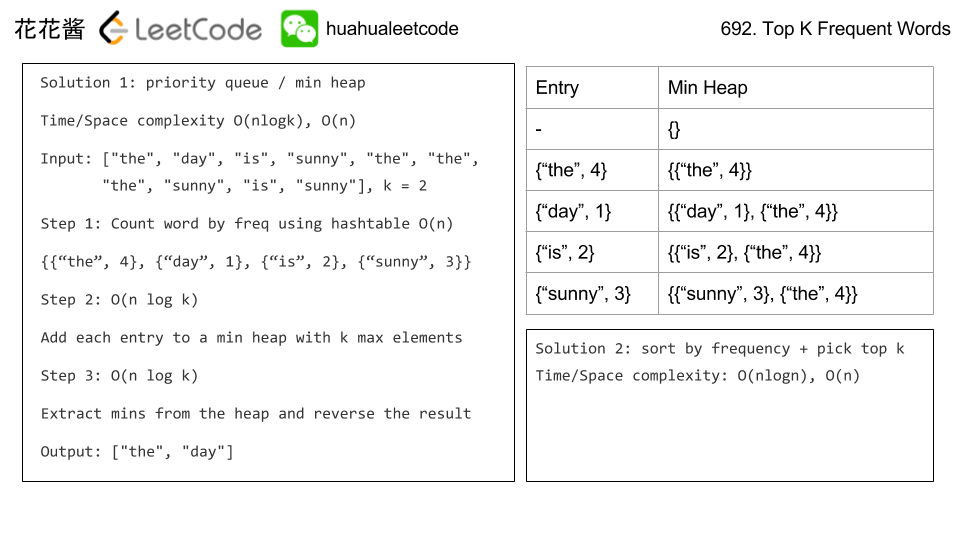Solution

C++ / priority_queue O(n log k) / O(n)

Related Problems

Problem:

A city’s skyline is the outer contour of the silhouette formed by all the buildings in that city when viewed from a distance. Now suppose you are given the locations and height of all the buildings as shown on a cityscape photo (Figure A), write a program to output the skyline formed by these buildings collectively (Figure B).

The geometric information of each building is represented by a triplet of integers [Li, Ri, Hi], where Li and Ri are the x coordinates of the left and right edge of the ith building, respectively, and Hi is its height. It is guaranteed that 0 ≤ Li, Ri ≤ INT_MAX0 < Hi ≤ INT_MAX, and Ri - Li > 0. You may assume all buildings are perfect rectangles grounded on an absolutely flat surface at height 0.

For instance, the dimensions of all buildings in Figure A are recorded as: [ [2 9 10], [3 7 15], [5 12 12], [15 20 10], [19 24 8] ] .

The output is a list of “key points” (red dots in Figure B) in the format of [ [x1,y1], [x2, y2], [x3, y3], ... ] that uniquely defines a skyline. A key point is the left endpoint of a horizontal line segment. Note that the last key point, where the rightmost building ends, is merely used to mark the termination of the skyline, and always has zero height. Also, the ground in between any two adjacent buildings should be considered part of the skyline contour.

For instance, the skyline in Figure B should be represented as:[ [2 10], [3 15], [7 12], [12 0], [15 10], [20 8], [24, 0] ].

Notes:

• The number of buildings in any input list is guaranteed to be in the range [0, 10000].
• The input list is already sorted in ascending order by the left x position Li.
• The output list must be sorted by the x position.
• There must be no consecutive horizontal lines of equal height in the output skyline. For instance, [...[2 3], [4 5], [7 5], [11 5], [12 7]...] is not acceptable; the three lines of height 5 should be merged into one in the final output as such: [...[2 3], [4 5], [12 7], ...]

Idea:

Sweep line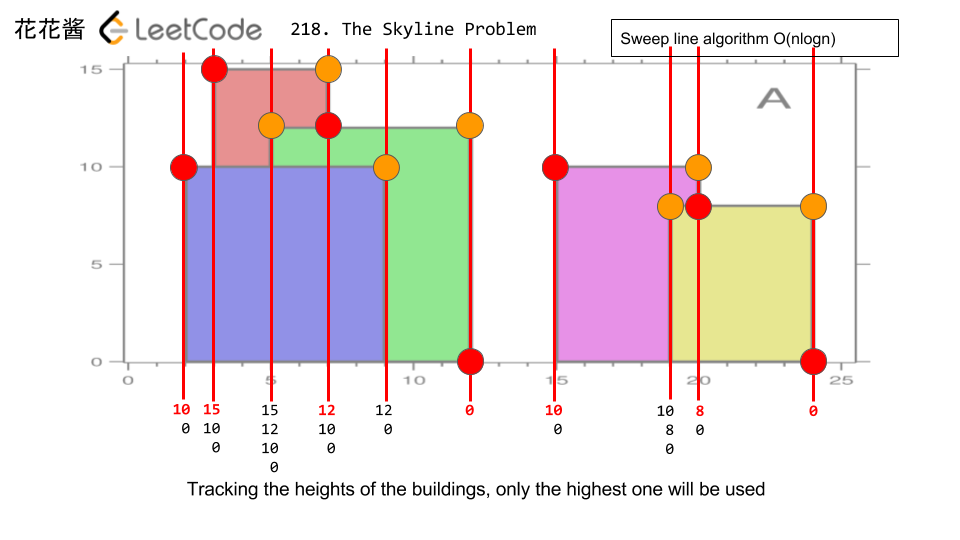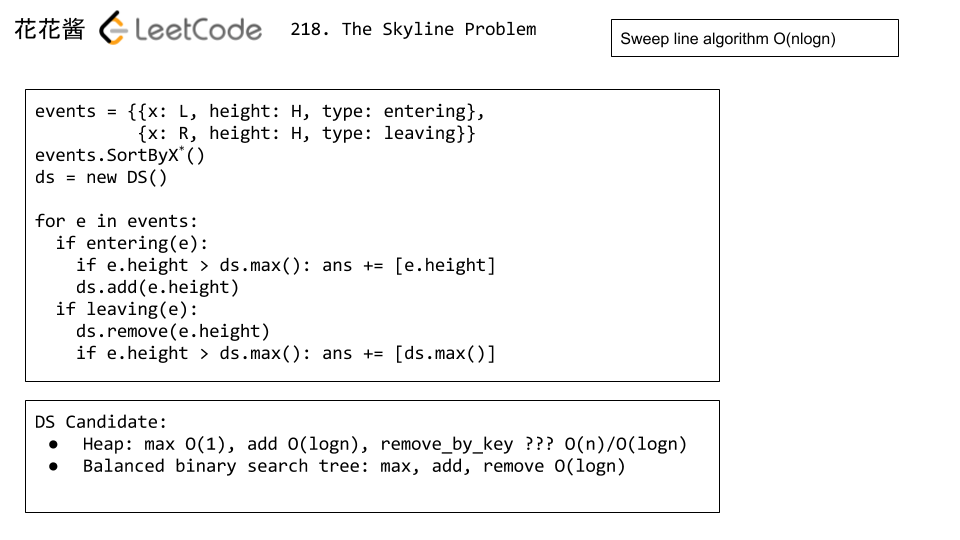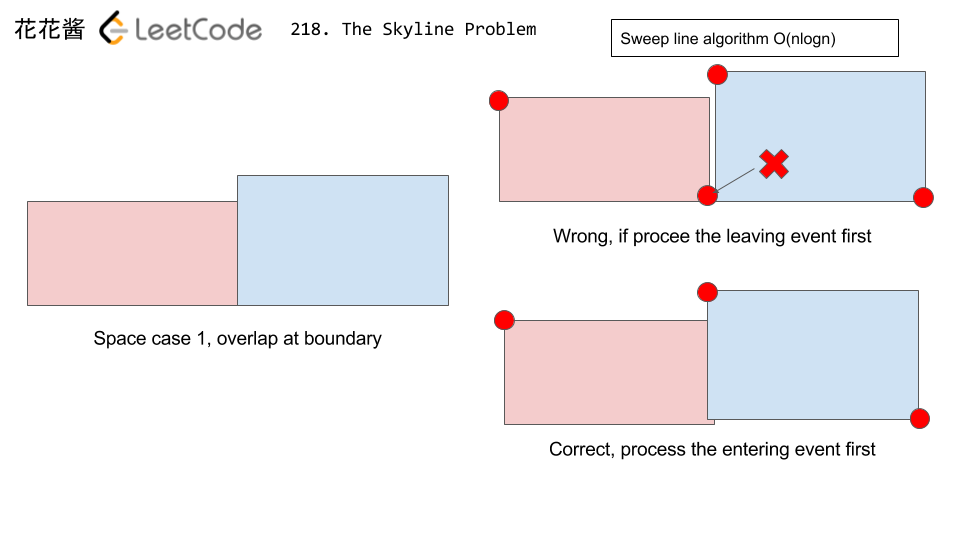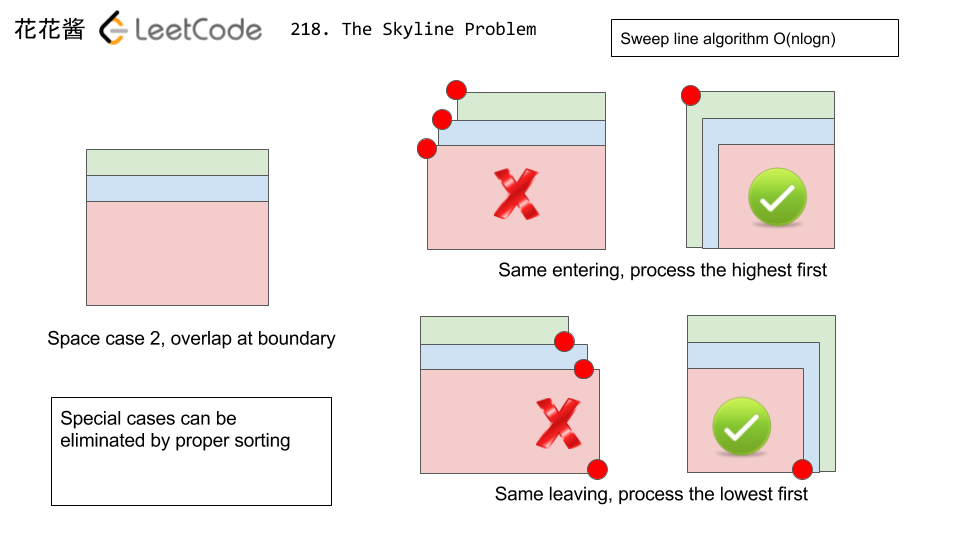Time Complexity:

O(nlogn)

Space Complexity:

O(n)

# Solution 2: Multiset

## C++

Problem:

Median is the middle value in an ordered integer list. If the size of the list is even, there is no middle value. So the median is the mean of the two middle value.

Examples:

[2,3,4] , the median is 3

[2,3], the median is (2 + 3) / 2 = 2.5

Design a data structure that supports the following two operations:

• void addNum(int num) – Add a integer number from the data stream to the data structure.
• double findMedian() – Return the median of all elements so far.

For example:

Idea:

1. Min/Max heap
2. Balanced binary search tree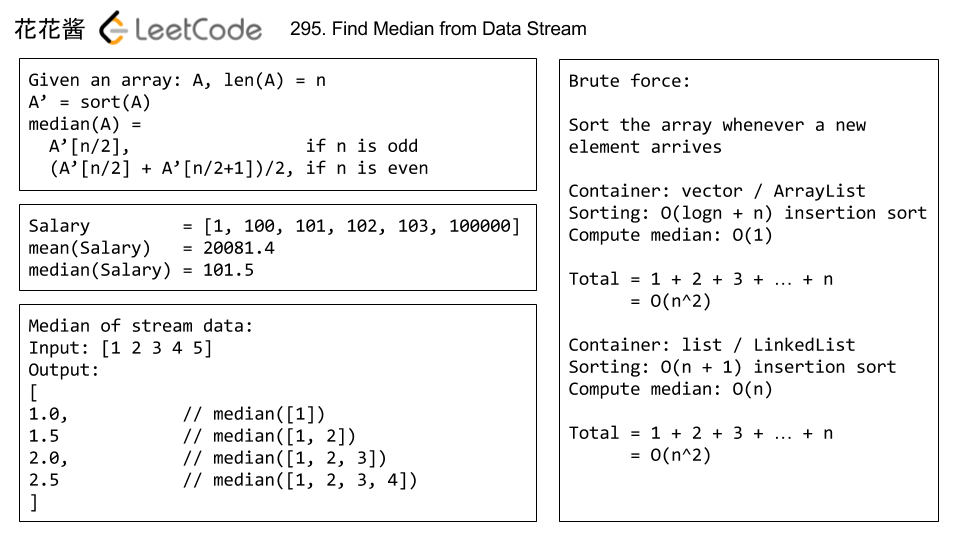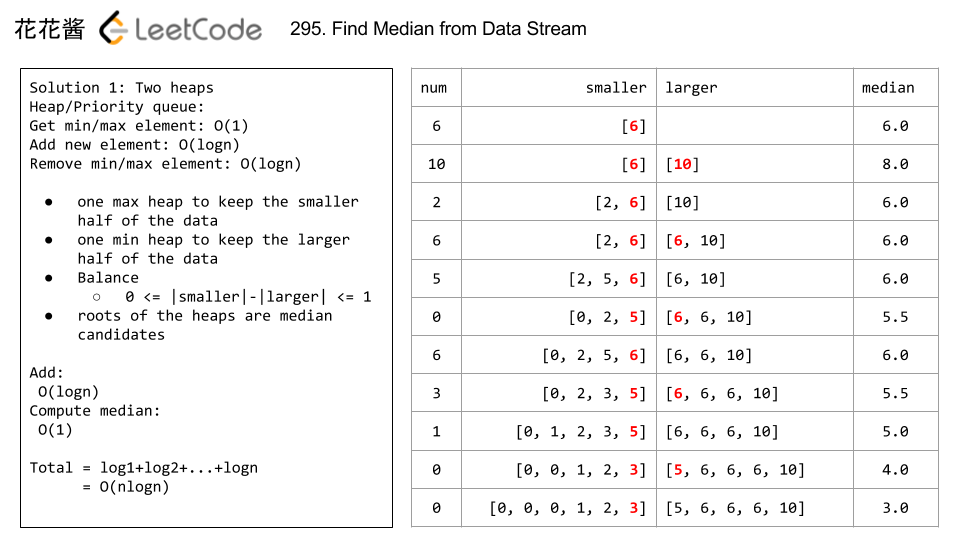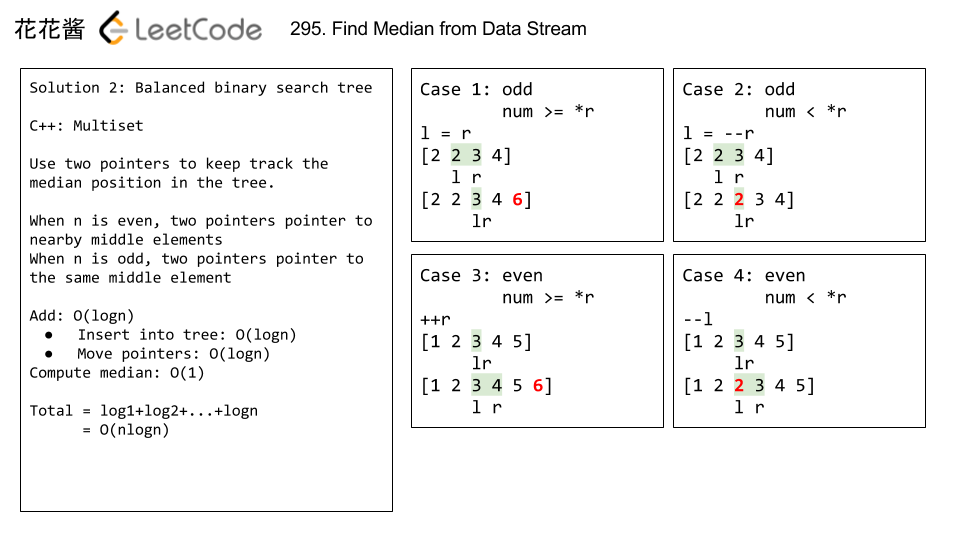Time Complexity:

findMedian(): O(logn)

Solution1:

Solution 2:

Related Problems

Mission News Theme by Compete Themes.